Posts

# How to Read an RxJS Marble Diagram

To an experienced RxJS user, marble diagrams are helpful. To someone just starting out with RxJS, they look like a little kid’s drawing in art class.

I’m by no means an expert user of RxJS, but I’ve used it enough to make sense of these “marble diagrams”. I can finally use rx marbles with confidence, and I want you to get there too.

*Note: this post assumes you are comfortable with the concept of asynchronous programming and Observables.*

## Let’s start simple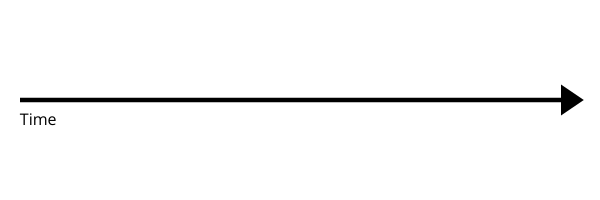Observables are asynchronous operations, so we need a way to represent the passage of time. This can be done with an arrow moving left to right.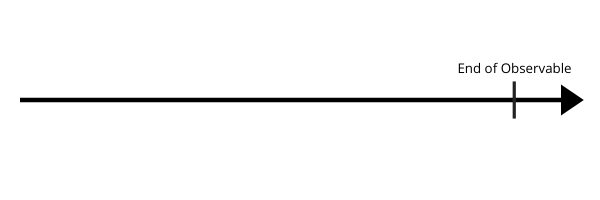The vertical line at the end of an arrow represents the successful completion of an Observable. But what if the Observable has an error?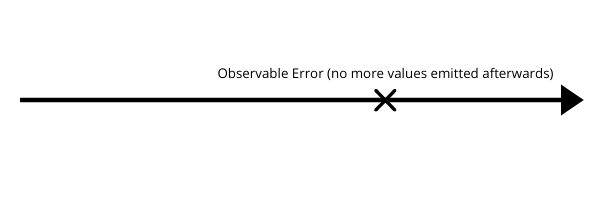If an error occurs in an Observable, it is represented by an X. Once an error is emitted, the Observable does not emit any further values.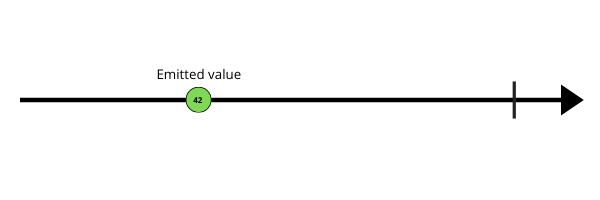And finally, those colorful little circles represent values and can show up anywhere on the arrow’s timeline. These values can be strings, numbers, booleans, or any other basic type.

## Putting the pieces together

Remember, marble diagrams help us understand operators. And operators come in two forms:

1. Creation operators (of, from, timer, etc.)

2. Pipeable operators (map, take, filter, etc.)

Creation operators are standalone (they create their own values), which means their marble diagrams are just a single arrow:_

And pipeable operators need an “Input Observable” as their source because they do not emit values themselves. They simply “operate on” those values. Therefore, you’ll see pipeable operator marble diagrams with 1 or more “Input Observables”, the operator itself, and an “Output Observable”.

Just think of these like normal functions (technically “pure functions”) except their arguments are observables and their return values are observables.

Here’s an example:It is important to note that the order of the input Observables matters in some cases. While some operators would return the same output Observable regardless of the order of the two input Observables, some operators actually use the order of those inputs to form the output. The above Observable `concat()`is a perfect example of this. Notice how the output Observable returns the three values emitted from input Observable #1 before returning the two values emitted from input Observable #2 even though both of Observable #2’s values were emitted prior to the final two values of Observable #1.

In RxJS, we generally refer to input Observable #1 as the “Outer Observable” and input Observable #2 as the “Inner Observable”.

As I said, the order doesn’t always matter. Take the `merge()` operator for example:No matter what order the two input Observables are called, the output Observable will always emit the same values (trust me for now — we’ll learn to read these in a few minutes).

## Pause: Let’s make sure we’re clear on a few things

To understand this post going forward, you need to get clear with some terminology:

Outer Observable: The outer Observable, or what I have called “input Observable #1”, or “OO”, is the Observable that is at the top of each diagram. It is called “outer” because it usually appears that way when writing code:

// An example that shouldn't make sense yet to you outerObservable().pipe( mergeMapTo( innerObservable(), (x, y) => x + y ) );

Inner Observable: The inner Observable, or what I have called “input Observable #2”, or “IO”, is the Observable below the outer Observable, but before the operator in each diagram. It is called “inner” for the same reason as above.

Output Observable: When using RxJS operators, sometimes there are many layers between the input Observable(s) and the output Observables, but you can think of the output Observable as the “return value”.

input Observable: This is a general term to identify *any *Observable that is NOT the “output Observable”. In other words, both the inner and outer Observables are considered “input” Observables.

And lastly, not all operators follow the concept of “inner” and “outer” Observables. To some operators like `combineLatest` (we’ll see this later), all Observables are treated equally, and therefore, we refer to each Observable as an “input Observable”.

## Let’s begin translating a few of these

Below are some of the most common operators and how to translate their marble diagrams.

We’ll start easy with the `map()` operator.The top arrow represents our input Observable, and it emits three values. This one is pretty straightforward if you’ve worked with the `map` function on a basic JavaScript array. All you’re doing is transforming the values emitted from the input Observable by a factor of 10. Here is the marble diagram recreated in code:We’ll do one more simple one and then jump into some harder ones. Below is the `take()` operator.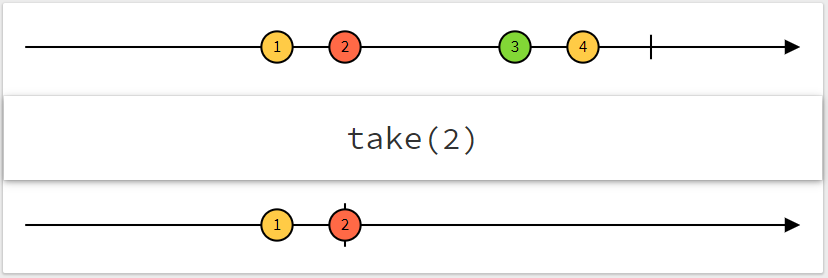In the above diagram, the input Observable emits four integers — 1, 2, 3, and 4. If you were to subscribe to this input Observable directly, you would receive those exact four values. But if you pipe the `take(2)` operator, the new output Observable will grab the first two emitted values, and then it will complete. The input Observable will still emit the last two values, but our output Observable will not see them because it has completed after two values. Below is the code and visualization.## switchMap Operator

Let’s look at some more difficult operators now. We’ll start with `switchMap()` as this is a common, yet sometimes difficult to understand operator.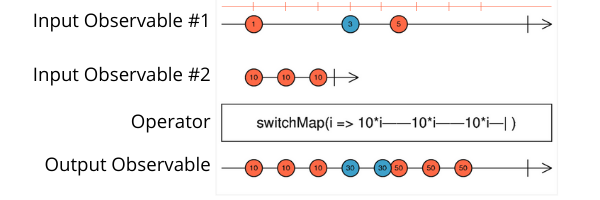And this is where these diagrams start getting a bit confusing, but by walking through each emitted value, we can start to make sense of it.

But before we go into that, let me highlight a few things.

• Notice that in this marble diagram, there are *two input Observables, *which means the code that you will see below this explanation is a bit more confusing. With an operator like `switchMap()`, Input Observable #1 is called the “Outer Observable” and Input Observable #2 is called the “Inner Observable”

• This example is from the official RxJS documentation because the rx marbles example is outdated (as of the time of writing) and uses the deprecated resultSelector.

• Because this is an example from the official documentation, you will notice that in the operator, there are a few dashes “-” within the function. You’ll also notice the “|” at the end. These simply represent the passage of time just as we have seen in the actual arrows. The more dashes, the more time between the emitted values. As you can see, the first two values of the outer observable take longer to emit than the last and have more dashes.

Here’s the diagram again so you don’t have to keep scrolling up.Let’s assume that each orange vertical line at the top represents 1 second. This means that the outer Observable (OO) emits values at 1, 4, and 5.5 seconds while the inner Observable (IO) emits values every second for 3 seconds, starting immediately after subscription (time zero).

The first three output values (10, 10, 10) seem pretty easy. Just multiply 1 x 10 = 10 for each of them according to the operator logic. Based on these first three values, we could say that for each OO value, the IO emits all of its values.

This assumption seems to hold true for the first output value of 30… And the second output value of 30…

But shouldn’t it emit a final value of 30??

This is where the `switchMap` logic comes in. Every time the OO emits a value, the IO emits all of its values *unless *the OO emits a new value before the IO finishes emitting all of its values. We can see this when the OO emits a value of 5 and it appears that our last value of 30 is “canceled”.

Takeway: What the marble diagram above is attempting to convey is that the output Observable of a `switchMap` operator is dictated by the outer Observable’s emitted values. How would you know this by looking at the diagram? In this case, you probably wouldn’t. Sometimes marble diagrams are only meaningful in conjunction with the operator description within the documentation. Here’s what the official documentation states:

Projects each source value to an Observable which is merged in the output Observable, emitting values only from the most recently projected Observable.

Translated, the “source value” would be the outer Observable values, while the “most recently projected Observable” represents the inner Observable values.

To some, this may not be confusing. But to me, it is. That’s why I generally start with the marble diagram, use some deductive reasoning, and then confirm my understanding with the official documentation (or other online tutorials).

Another takeaway: There is no “formula” for interpreting marble diagrams. As you’ll see in subsequent examples, interpreting marble diagrams is kind of like solving a logic puzzle. By figuring out what the operator does not do, you will be able to figure out what it does (for you Taleb readers — “via negativa”).

Below is some code that attempts to replicate the diagram above (note that there are more efficient ways to write the inner and outer observables, but this was the clearest way I could think of).## Another example: combineLatest

Now that we have a basic idea of how to interpret a marble diagram, let’s practice another one — combineLatest.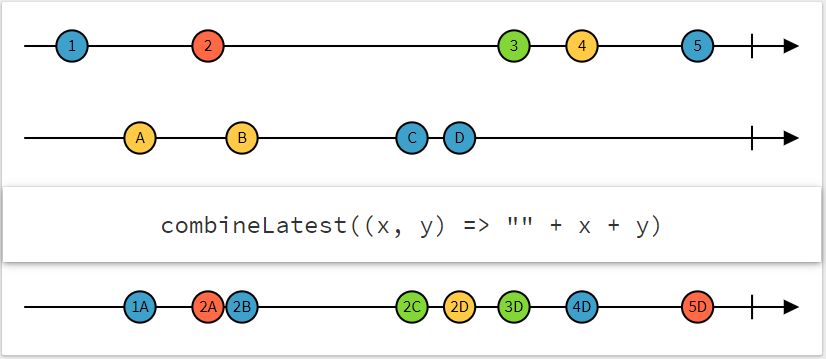Note: `combineLatest` doesn’t follow the concept of “outer Observable” and “inner Observable”. With this operator, all Observables are treated equally.

This is a good one because you can infer how it works without any descriptions. Starting with the first emitted value, you’ll notice that there is no output value.

This tells us that `combineLatest` requires both input Observables to emit at least one value before it emits the first output value.

The next emission comes from the second input Observable, and you’ll see that when it emits, we get our first output value, “1A”.

Clearly, this operator is combining the values from each input Observable (hence the name). But at this point, we don’t exactly know how yet.

Next up, the first input Observable emits the value 2, and we see that our output value is “2A”. It is obvious where the 2 came from, but where did the “A” come from? Well, consistent with its name, “A” was the latest value of the second input Observable.

Jumping to the last output value, “5D”, you can see that input Observable #1 emitted the value 5, and the latest emitted value from input Observable #2 was “D”.

## Let’s keep it rolling with the “filter” operator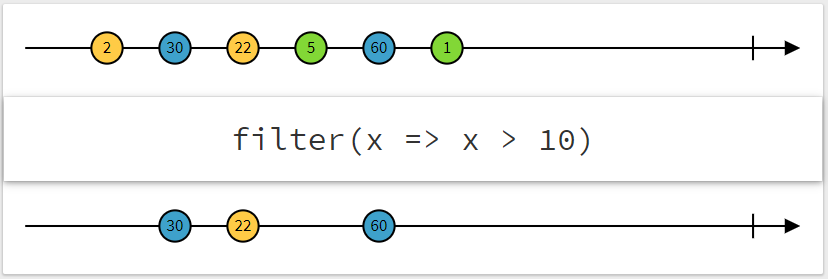We’re back to having only one input Observable. Starting with the first emitted value of 2, we see that there is no output value. Looking at the filter operator logic, we can see that we are looking for emitted values that are greater than 10. We can logically conclude that since the first emitted value was less than 10, it was ignored and there was no output emission.

By the second value, our understanding is confirmed. The second emitted value is 30, which is greater than 10, and we see that because of this, the output Observable emits the value.

## Another one: zip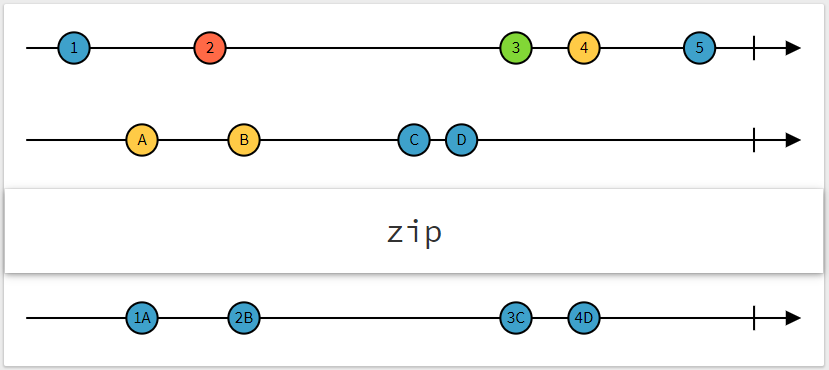Looks a lot like `combineLatest` right?

The first two output Observable values of “1A” and “2B” seem to suggest that `zip` works exactly like `combineLatest`. But once we get to the third output Observable value of “3C”, things don’t make sense anymore…

If I were reading this marble diagram having never used `zip` before, I would test out a few assumptions until my assumption held true for all the output values. My initial assumption might have been that this operator combines the latest values of the input Observables. This is proven false by the output value of “3C” because if it emitted the latest values, this should have been “3D”.

So my new assumption — the `zip` operator “matches up” values of each input Observable. For example, the fourth value of input Observable #1 should be combined with the fourth value of input Observable #2.

The fourth value of each Observable is 4 and “D”. Do we have an output value of “4D”?

Yes we do!

And the fifth value of each Observable is 5 for input Observable #1 and nothing for input Observable #2.

Since both input Observables don’t have a fifth value, there is nothing to “match up” and therefore no output value.

## Last One: mergeMapTo (challenging)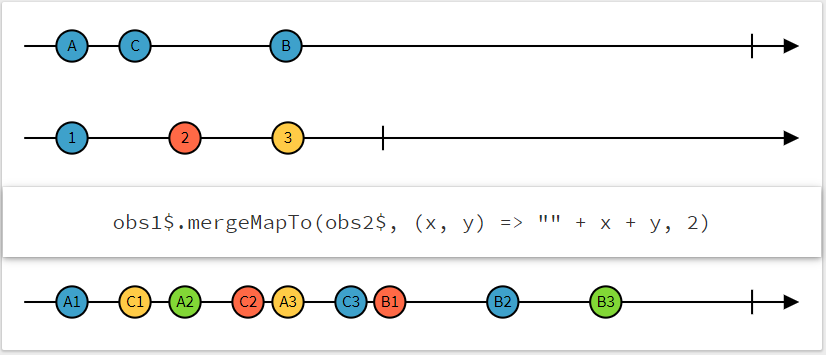Note: I chose this operator because it looked difficult. I have never actually used this operator in my code and don’t know of a good reason to (comment if you do!)

By scanning the operator logic, we can tell that the two input Observables are being combined into a single string value. So let’s use this to make our initial assumption as to how this operator works.

I see that the first output value combines both Observables. This doesn’t tell me much because there are several other operators that do the same (combineLatest, zip, etc.).

The next output is “C1”. This also doesn’t tell me much because `combineLatest` does the same thing…

The next output value is “A2”, and this is where we start building our assumption. If this operator were `combineLatest`, this output value should have been “C2” (the latest two values). If this operator were `zip`, the value should have been “B3”. So now, we must figure out what logic is happening to produce a value of “A2”.

This operator is called mergeMapTo (emphasis on “merge”), so it is probably additive. I also see a lot of output values. So a good assumption is that this operator is coming up with every possible combination of input Observable values. Let’s see if this holds true…

First, let’s list off all the possible combinations of values from the two input Observables:

A1, A2, A3, C1, C2, C3, B1, B2, B3

And do all of these values appear as output values? Yep.

So the last part of this equation is to figure out *how *this is being applied. Because with operators, the order and timing of things matters.

Above, I’ve listed all of the possible values using the two input Observable emissions. But the order which I listed them in is NOT the order which they were emitted in. Here is the order they were emitted:

A1, C1, A2, C2, A3, C3, B1, B2, B3

So the question is… Why did “C1” come before “A2”? And why did “A3” come after “C2”? There is clearly something going on with the order of emissions here.

Here’s the diagram again so you don’t have to keep scrolling up.I’m noticing a couple of things here:

• Each time either of the input Observables emit a value, the output Observable may, but doesn’t always emit a value (unlike `combineLatest`)

• The output Observable never emits a value that hasn’t been emitted by the input Observables yet

• The inner Observable (IO) is directly related to the output value. In other words, each time it emits a new value (1, 2, 3), the output Observable emits a value with that newly emitted value in it. For example, take a look at the output value “A3”. The outer Observable (OO) just emitted the value “B”, and the IO just emitted the value 3. But in this case, the output value doesn’t have “B” in it at all, which means that the output isn’t concerned with the timing of OO, but it *is *concerned with the timing of IO.

• The output values look random at first, but notice how “A1”, “A2”, and “A3” are spaced out evenly — the exact same spacing as the IO. This also occurs for “B1”, “B2”, and “B3”, and “C1”, “C2”, “C3”— they are spaced out evenly.

So with these observations, here is my conclusion as to how this operator logic works:

Each time the outer Observable (input Observable #1) emits a value, a new “sequence” (not a new Observable) is fired. This sequence is entirely based on the number of values and timing of values seen in the inner Observable (input Observable #2).

Here’s some code to represent the diagram for those interested:## There is more than one way to do it

Reading marble diagrams is more of an art than a science. Once you understand the components of a marble diagram, you can use deductive logic (as seen above), you could search the internet for tutorials on the specific operator you are using (probably quicker than deductive logic), or you could painfully learn to read the RxJS documentation and all of the jargon associated with that. Or… You could combine all of these approaches into one (my preference).

And as you can see, the more operators that you learn, the easier it is to learn new ones because you can start making associations between them and recognizing operators that are similar to one another.

## The Operator Decision Tree

And finally… Just because you can read marble diagrams does not mean that you need to learn every operator. While learning all the operators thoroughly would be extremely helpful, not all of us have the time to do so (and after using RxJS long enough, you’ll find yourself re-using the same few operators over and over again). And for that reason, here’s a workflow that I’ve used with RxJS:

1. Identify the problem you’re trying to address (aka you know you need an operator but aren’t sure which one)

2. Use the operator decision tree

3. Interpret the marble diagram of the operator that the decision tree chooses

4. If your interpretation of the operator seems like it addresses the problem from step #1, you’re done. If not, repeat these steps until you’ve narrowed it down enough.

## That’s it!

Here are some other articles of mine you might like:

Three things I wish I knew when I started using Angular 2+

The most confusing thing in JavaScript: The this keyword